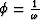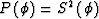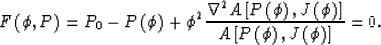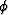Next: Evaluation of derivatives of Up: Biondi: Solving the frequency-dependent Previous: FREQUENCY-DEPENDENT EIKONAL

# SOLVING THE FREQUENCY-DEPENDENT EIKONAL

The frequency-dependent eikonal equation (4) cannot be solved separately from the transport equation because the phase slowness is dependent on the amplitudes. An exact numerical solution of the combined equations is difficult to compute. I therefore investigate a method for computing an approximate solution. My method extrapolates the phase-slowness function along the frequency axis, starting from infinite frequency where the phase slowness function is equal to the medium slowness. This scheme assumes that the phase slowness is a continuous function of frequency, and that it can be extrapolated in frequency by solving a partial differential equation. After the phase slowness function is computed, the traveltimes and the amplitudes can be computed at each frequency by using conventional dynamic raytracing Cervený (1987).

To extrapolate the phase slowness I derive from equation (4) an expression for its derivative with respect to frequency. I start by rewriting equation (4) in terms of the inverse of the frequencyand the square of the slowness,(8)
The derivative ofwith respect tois found by applying the rule of implicit differentiation to the function(9)
Equation (9) is a non-linear partial differential equation, that can be solved by explicitly stepping in,starting from the initial value.For each value of, and corresponding value of,the right hand side of the equation can be evaluated and used for computing the value of the phase slowness for the next. A variety of explicit integration schemes can be used for integrating equation (9); I used a standard second-order Runge-Kutta method.

Because equation (9) is non-linear, the analysis of convergence and stability of its numerical solutions is not trivial, and I have not performed it yet. However, the numerical experiments that I have run, and that I will describe in the next section, show that the solution of equation (9) leads to satisfactory results. The solution is also stable, if the round-off errors for the high-wavenumbers components of the Laplacian of the phase slowness are adequately constrained.Next: Evaluation of derivatives of Up: Biondi: Solving the frequency-dependent Previous: FREQUENCY-DEPENDENT EIKONAL
Stanford Exploration Project
11/18/1997